# Cents

Musical intervals are often expressed in cents, a unit of pitch based upon the equal tempered octave such that one equal tempered semitone is equal to 100 cents. An octave is then 1200˘ and the other equal tempered intervals can be obtained by adding semitones: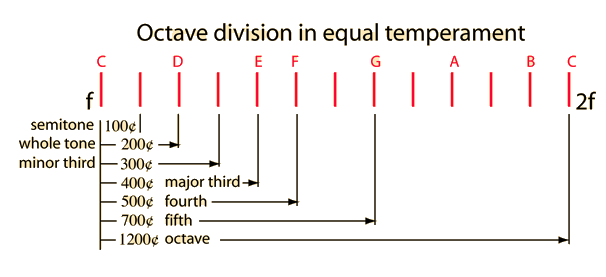If f1 = Hz and f2 = Hz then the interval is cents.

 Advantages of cents Mathematical expressions Just noticeable difference in pitch
Index

Temperament and musical scales

 HyperPhysics***** Sound R Nave
Go Back

# Calculating Cents

The fact that one octave is equal to 1200 cents leads one to the power of 2 relationship: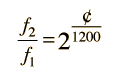This is convenient for calculating the frequency corresponding to a certain number of cents. To calculate the number of cents for any two frequencies, the above relationship must be reversed. Taking the log of both sides gives: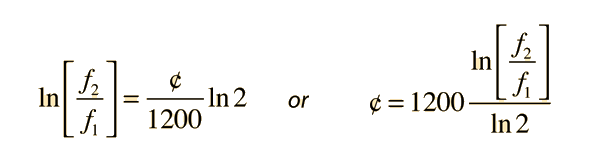If f1 = Hz and f2 = Hz then the interval is cents.

Index

Temperament and musical scales

 HyperPhysics***** Sound R Nave
Go Back

Examining the semitone A to B-flat at different points in the range of the piano will illustrate the fact that if expressed in cents, every equal tempered semitone is the same. Expressed in Hz difference, every semitone is different. The interval value in cents expresses the ratio of the frequencies, which is the same for every equal tempered semitone.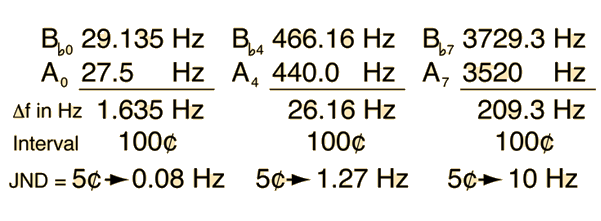Included with the semitone intervals above is an evaluation of the deviation in Hz needed to equal 5˘, the nominal just-noticeable difference for these pitches. Note that the range represented by 5˘ increases from less than a tenth of a Hz at the low end of the piano to about 10 Hz at the top end of the piano.

 If f1 = Hz and f2 = Hz then the interval is cents.

 Just noticeable difference in cents
Index

Temperament and musical scales

 HyperPhysics***** Sound R Nave
Go Back

# Just Noticeable Difference in Pitch

The just noticeable difference in pitch must be expressed as a ratio or musical interval since the human ear tends to respond equally to equal ratios of frequencies. It is convenient to express the just noticeable difference in cents since that notation was developed to express musical intervals. Although research reveals variations, a reasonable estimate of the JND is about five cents. One of the advantages of the cents notation is that it expresses the same musical interval, regardless of the frequency range.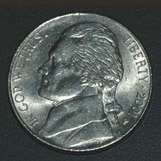You can hear about a nickel's worth of difference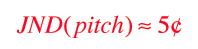More detail
Index

Temperament and musical scales

Reference
Rossing
Science of Sound, 2nd ed.

 HyperPhysics***** Sound R Nave
Go Back

# Pitch Details Related to Cents

Evaluating the just noticeable difference in pitch by the "nickel's worth" rule is convenient, but as you might expect it is an oversimplification. Rossing describes measurements of pitch discrimination with pure tones at about 80 dB for frequencies between 1 and 4 kHz. The jnd is found to be about 0.5% of the pure tone frequency, which corresponds to about 8˘ . He also states that the jnd has been found to depend upon the frequency, the sound level, the duration of the tone, the suddenness of the frequency change, the musical training of the listener, and the method of measurement. The real world can rarely be accurately characterized by simple rules.

Rossing also reports that the critical bandwidth (related to loudness perception) is about 30 times the jnd for pitch, suggesting that both are related to the regions of excitation along the basilar membrane of the inner ear.

 Frequency intervals in cents
Index

Temperament and musical scales

Reference
Rossing
Science of Sound, 2nd ed.

 HyperPhysics***** Sound R Nave
Go Back